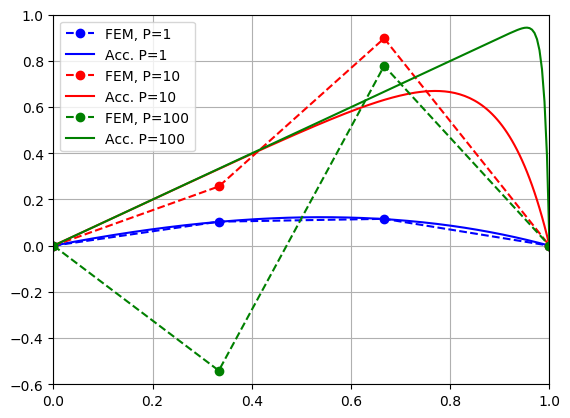In last example, a very basic 1d problem of type $u'' = f$ was constructed. In this Julia programming example a new FEM calculations is done to solve diffusion-convection equation $au'' + bu' = f$.

While it first look that this is just a minor change to what is done before, a lot of new concepts is introduced about programming the finite element method: $a$ and $b$ are introduced as a field variables and numerical integration is performed over domain using Gaussian quadratures.

The resulting convective term is causing unsymmetric stiffness matrix and it can be seen that solution is unstable without introducing numerical dissipation, which is interesting from theory point of view. More information about this can be found from Abaqus documentation and other literature references listed at end of this post.

The main lesson to learn is that implementing new features to dynamically typed open source finite element method softwares like JuliaFEM is not hard task and is not taking more than 20 lines of code. Julia code itself has a very clear and intuitive syntax and learning Julia programming language is easy.

Because "input file" of JuliaFEM is Julia programming language script itself, all programming concepts are available for complicated design processes involving optimization loops and communication with other softwares. It's also possible to use other Julia packages and they can be called from the same script which is used to run simulation.

We start like before, defining a new problem which is a subtype of FieldProblem and define some name for the field we are solving, let's that be $u$:

In :
using JuliaFEM
import JuliaFEM: assemble!, get_unknown_field_name

In :
type DiffusionConvection <: FieldProblem
end

In :
function get_unknown_field_name(problem::Problem{DiffusionConvection})
return "u"
end

Out:
get_unknown_field_name (generic function with 6 methods)

In assemble!, we first initialize local matrices and then loop over integration points of element.

$a$ and $b$ are "fields" which are updated to element before assembly operation. Duct typing is used here: we don't really care what $a$ and $b$ are as long as they are returning something numerical in element domain for $\xi$ for some time $t$.

Finally we just add all evaluated quantities to local stiffness matrix $K_e$ and force vector $f_e$, note the unsymmetry in convective term.

In :
function assemble!(assembly::Assembly,
problem::Problem{DiffusionConvection},
element::Element{Seg2}, time)
Ke = zeros(2, 2)
fe = zeros(2)
for ip in get_integration_points(element)
detJ = element(ip, time, Val{:detJ})
a = element("a", ip, time)
b = element("b", ip, time)
N = element(ip, time)
Ke += ip.weight * a*dN'*dN * detJ
Ke += ip.weight * b*N'*dN * detJ
fe += ip.weight * N' * detJ
end
gdofs = get_gdofs(problem, element)
end

Out:
assemble! (generic function with 36 methods)

Next we define a small helper function taking number of nodes and Peclet number as input arguments, so that we can study the effect of Peclet number to the solution. In short, we define calculating grid for $X \in [0, L]$ for $N$ nodes, create elements, update fields geometry, a and b, define new problem, add elements to problem, assemble global matrices and solve system $Ku=f$.

Boundary conditions $u(0)=0$, $u(L)=0$ are taken into account by removing the first and last dof from solution. Of course we have methods to define boundary conditions like Problem{Dirichlet} and solver structure Solver{Linear} to solve this kind of problem, but let's keep things simple at this point. And I think it's interesting to see what is happening under the hood because this is more like development example, not usage example.

In :
function get_sol(N=4, P=1.0)
grid = linspace(0, 1.0, N)
X = Dict(i => [x] for (i, x) in enumerate(grid))
elements = [Element(Seg2, [i, i+1]) for i=1:N-1]
update!(elements, "geometry", X)
update!(elements, "a", 1.0)
update!(elements, "b", P)
problem = Problem(DiffusionConvection, "test problem", 1)
initialize!(problem)
assemble!(problem)
K = sparse(problem.assembly.K)
f = full(problem.assembly.f)
u = zeros(N)
u[2:end-1] = lufact(K[2:end-1,2:end-1]) \ f[2:end-1]
return grid, u*P
end

Out:
get_sol (generic function with 3 methods)
In :
L1, u1 = get_sol(4, 1.0)
L2, u2 = get_sol(4, 10.0)
L3, u3 = get_sol(4, 100.0)

16-Sep 23:53:43:WARNING:root:assemble!() this is default assemble operation, decreased performance can be expected without preallocation of memory!
16-Sep 23:53:44:WARNING:root:assemble!() this is default assemble operation, decreased performance can be expected without preallocation of memory!
16-Sep 23:53:45:WARNING:root:assemble!() this is default assemble operation, decreased performance can be expected without preallocation of memory!

Out:
(0.0:0.3333333333333333:1.0, [0.0, -0.540826, 0.778261, 0.0])

We get into the warnings in next posts.

Visualization of solutions with different Peclet numbers can be done e.g. using PyPlot, what is old good matplotlib wrapped to Julia programming environment.

In :
using PyPlot
x = linspace(0, 1, 200)
u_acc(P) = x - exp.(-P*(1-x)) .* (1-exp.(-P*x)) / (1-exp.(-P))
p = plot(L1, u1, "--bo", label="FEM, P=1")
plot(x, u_acc(1.0), "-b", label="Acc. P=1")
plot(L2, u2, "--ro", label="FEM, P=10")
plot(x, u_acc(10.0), "-r", label="Acc. P=10")
plot(L3, u3, "--go", label="FEM, P=100")
plot(x, u_acc(100.0), "-g", label="Acc. P=100")
ylim(-0.6, 1.0)
xlim(0.0, 1.0)
grid()
legend()Out:
PyObject <matplotlib.legend.Legend object at 0x000000004F7055F8>

Here we can observe that when the convective term with respect to diffusion term increases, solution is unstable. Indeed there is a way to make formulation stable by adding an artificial diffusion term, but they are offtopic of this example. More information about this technique can be found from [1, p. 59].

1. Kouhia, Reijo. Rakenteiden mekaniikan numeeriset menetelmät.
2. Abaqus Documentation. 2.11.3 Convection/diffusion.